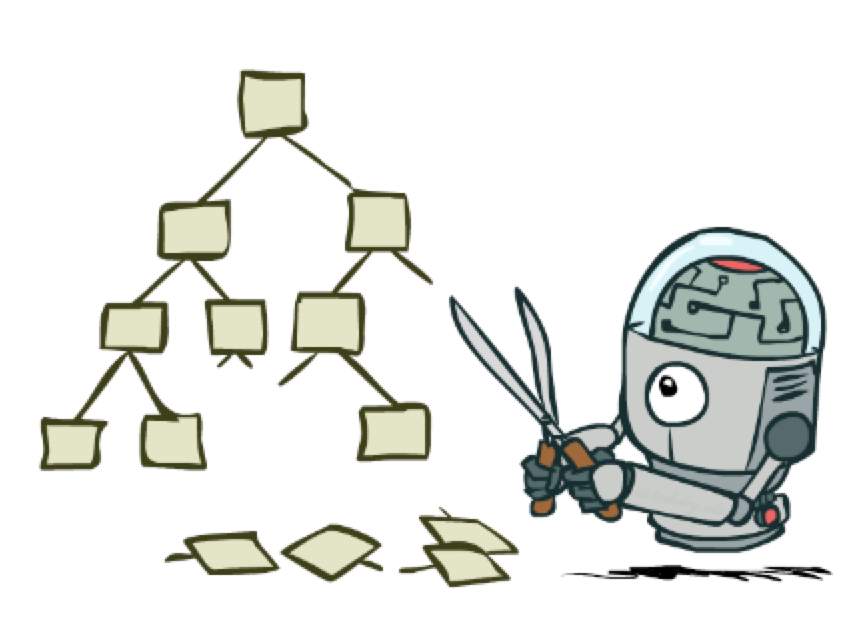CS 440/ECE 448
Margaret Fleck

## Games 4from Berkeley CS 188 slides

## Recap: minimax search

Recall that our basic minimax search algorithm looks like this:

• Do recursive depth-first search, with depth limit d.
• When we hit a node at depth d, use heuristic function to evaluate the game position.
• As we recurse upwards, use minimax to propagate values upwards
• Pick best move available to us at the root node

## Pseudocode for minimax

In order to discuss the details of alpha-beta pruning, we need to write detailed pseudocode for minimax. In building out our search tree, we create the children of a node n by taking an action, e.g. moving a piece in a chess game. Let's define:

• move(n,a) is the child of n that results from taking action a
• the "value" of a node is either its actual value (if known) or a heuristic estimate (if we've reached the depth limit)

Minimax is usually implemented using two mutually recursive functions, min-value and max-value. The input to min-value should be a min node and min-value returns the minimax value for that node. The function max-value does the same thing for an input max node.

max-value (node)

• if node is a leaf, return its value
• else
• rv = -inf
• for each action a
• childval = min-value(move(node,a))
• rv = max(rv, childval)
• return rv

min-value (node)

• if node is a leaf, return its value.
• else
• rv = +inf
• for each action a
• childval = max-value(move(node,a))
• rv = min(rv, childval)
• return rv

The above functions compute the value for the top node in the game tree. That will be convenient for discussing alpha-beta pruning. However, it's not actually what you'll do if you are implementing a game player. A game player needs his next move, not the value for the root node of the tree (aka the current game state). So our game playing code starts with a modified function at the top level which does this:

Choose-move(n)

• Create nodes for all children of n.
• Use min-value to compute the value for each child node.
• Pick the action corresponding to the child with the best value.

Detailed code for choose-move is essentially similar to max-value, except that it returns argmax rather than max.

## What are alpha and beta?

To add alpha-beta pruning to our minimax code, we need two extra variables named (big surprise!) $$\alpha$$ and $$\beta$$. The algorithm passes $$\alpha$$ and $$\beta$$ downwards when doing depth-first search. We'll use $$\alpha$$ and $$\beta$$ to propagate information from earlier parts of our search to the path that we're currently exploring.

Suppose that our search is at some node n. There may be a number of paths leading from the root downwards to a leaf, via n. The "outcome" of a path is the value that it sends upwards to the root (which might not be the best value that arrives at the root).

• $$\alpha$$ is a lower bound on the outcome for paths through n
• $$\beta$$ is an upper bound on the outcome for paths through n

The values of $$\alpha$$ and $$\beta$$ are based partly on values from descendents of the current node n. However, they are also based on values from already-explored nodes to the left of n. When nodes to the left of n have already found a better value for one of n's ancestors, we can stop exploring the descendents of n. The word "better" might translate into either larger or smaller, depending on whether the ancestor is a max or min node.

Suppose that v(n) is the value of our current node n (based on the children we have examined so far). If there's still a chance that the best path goes through n, we must have

$$\alpha \le v(n) \le \beta$$

As a node examines more and more of its children

• A max node can increase $$\alpha$$
• A min node can decrease $$\beta$$

We return prematurely if either of the following two situations holds:

• $$v(n) > \beta$$ or $$v(n) < \alpha$$   (No viable path through node n.)
• $$v(n) = \beta$$ or $$v(n) = \alpha$$   (No path through node n can improve on what we've already found.)

## Pseudocode for alpha-beta

Here's the pseudo-code for minimax with alpha-beta pruning. The changes from normal minimax are shown in red. Inside the inner loop for max-value, notice that rv gradually increases, as does alpha. The function returns prematurely if rv reaches beta.

max-value (node, alpha, beta )

• if node is a leaf, return its value.
• else
• rv = -inf
• for each action a
• childval = min-value(move(node,a), alpha, beta )
• rv = max(rv, childval)
• if rv >= beta, return rv    # abandon this node as non-viable
• else alpha = max(alpha, rv)
• return rv

Similarly, inside the inner loop for min-value, rv gradually decreases and so does beta. The function returns prematurely if rv reaches alpha.

min-value (node, alpha, beta )

• if node is a leaf, return its value.
• else
• rv = +inf
• for each action a
• childval = max-value(move(node,a), alpha, beta)
• rv = min(rv, childval)
• if rv <= alpha, return rv    # abandon this node as non-viable
• else beta = min(beta,rv)
• return rv

Yes, it's very easy to get parts of this algorithm backwards. We're not going to ask you to produce this level of detail on an exam. Concentrate on understanding what branches will be pruned, as shown in the previous lecture. But it's worth seeing the pseudocode briefly so that you can feel confident that alpha-beta pruning isn't complicated if you ever need to implement it.